## GMAT Question Analysis #1

For this week’s post I decided to do a GMAT math question. The Official Guide for the GMAT provides explanations for all included questions, so I’m going to write one of my own and discuss it. This question could just as easily show up on a test like the GRE, the SAT, or the ACT.

The number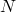is increased by% and then the result is decreased by%. If the result is% ofwhich of the following could be the value of?

A.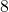B.C.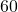D.E. None of these

…And we’re back!

This question tests on the concept of percent change in a somewhat tricky roundabout fashion.

At first blush, it might seem like we’re asked to do the impossible here and that the answer must be E. Shouldn’t increasing and then decreasing by% leave us exactly where we started? In fact, this is not the case, because the% increase is taken on the original number, whereas the% decrease is taken on the subsequent increased value. Increasing and then decreasing by a given percent will always result in a value lower than before. (Decreasing and then increasing by% would be no different.)

In order to solve this problem, we translate the given statements to algebra. Saying that “the numberis increased by%” means thatis increased by%… of. So%is what we want — don’t get caught writing% instead. Factoring, we get%. We could go a step further by recognizing that “percent” literally means “divided by.” Thus,%is the same as. Either way, the takeaway point is that increasing a number by% is the same as multiplying that number by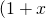%.

Analogously, decreasing a number by% is the same as multiplying that number by%.

Putting these operations together, we get:%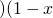%%Cancellingfrom both sides and noting that % means “divided by,”Multiplying both sides by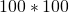to cancel the fractions,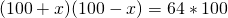This is a difference of squares;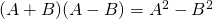, so: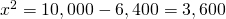So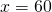or, and the answer is C.

Bonus Question:

The numberis increased by% and then the result is decreased by%. If the result iswhich of the following is the value of, in terms ofand?

A.B.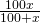C.D.E.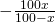Okay, let’s jump right in. The first statement, “is increased by%,” means that we have. The second statement, “the result is decreased by%,” means that our prior result,, is multiplied by; the result,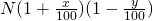is equal to. Solve foras follows: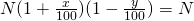Dividing both sides byeliminates that variable entirely. The answer will not depend on.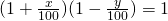Let’s multiply through by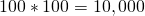to eliminate the fractions.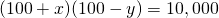Next, divide by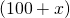, subtract, and then multiply by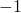to isolate:A look at the answer choices shows that a common denominator is needed here. That common denominator is.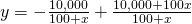The answer is B.

Tags: , , , , , , ,